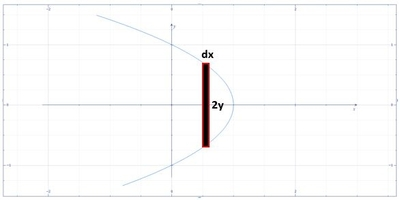# Find the volume of a solid S in which the base of the S is the region enclosed by the parabola x...

## Question:

Find the volume of a solid {eq}S {/eq} in which the base of the {eq}S {/eq} is the region enclosed by the parabola {eq}x = 1 - y^2 {/eq} and the {eq}y {/eq}-axis. Cross-sections perpendicular to the {eq}{/eq}-axis are semicircles.

## Volume of the Solid:

The problem is about solving the volume of the solid using the slicing method and note that in this method the formula we use for volume is {eq}V=A\times l {/eq} where {eq}A {/eq} is the area of the cross-section and {eq}l {/eq} is the length. Here, since the cross-section is a semicircle thus its area is {eq}A=\frac{\pi }{8}d^{2} {/eq} where {eq}d {/eq} is the diameter.

## Answer and Explanation:

Below is the graph,From the graph,

{eq}dV=\left [ \frac{\pi }{8}\left ( 2y \right )^{2} \right ]dx {/eq}

{eq}V=\frac{\pi...

See full answer below.

Become a Study.com member to unlock this answer! Create your account

#### Learn more about this topic:Volumes of Shapes: Definition & Examples

from GMAT Prep: Tutoring Solution

Chapter 11 / Lesson 9
45K